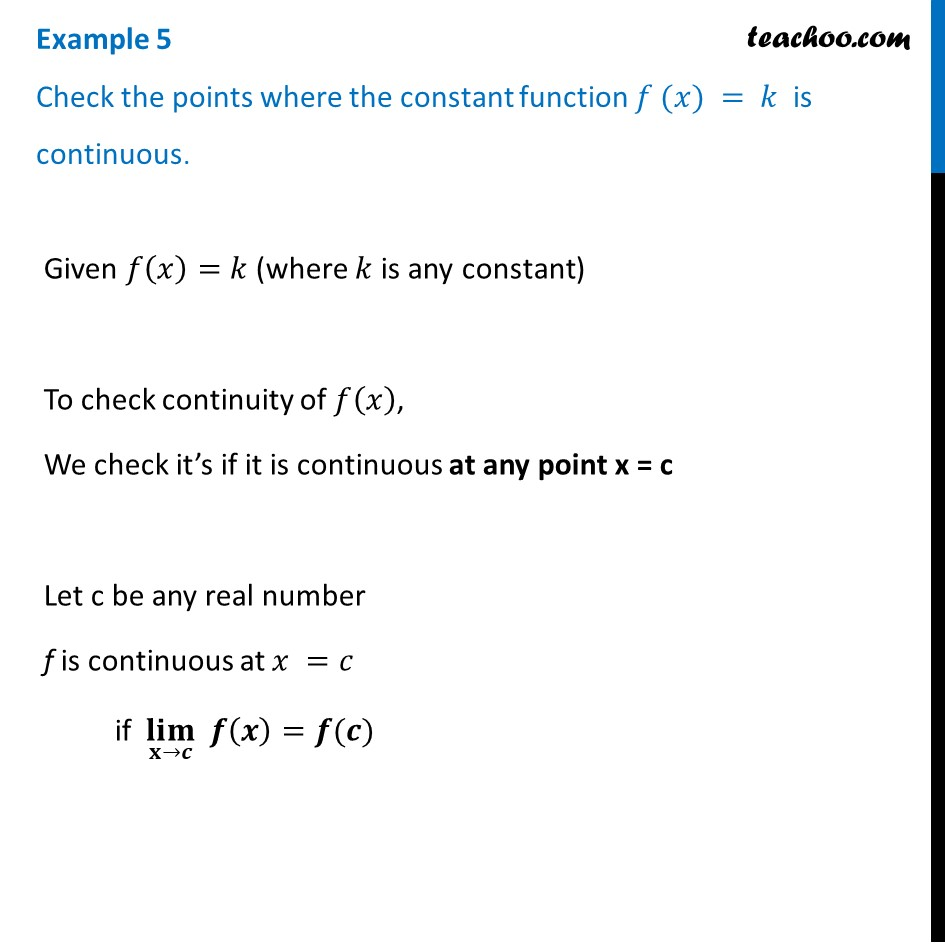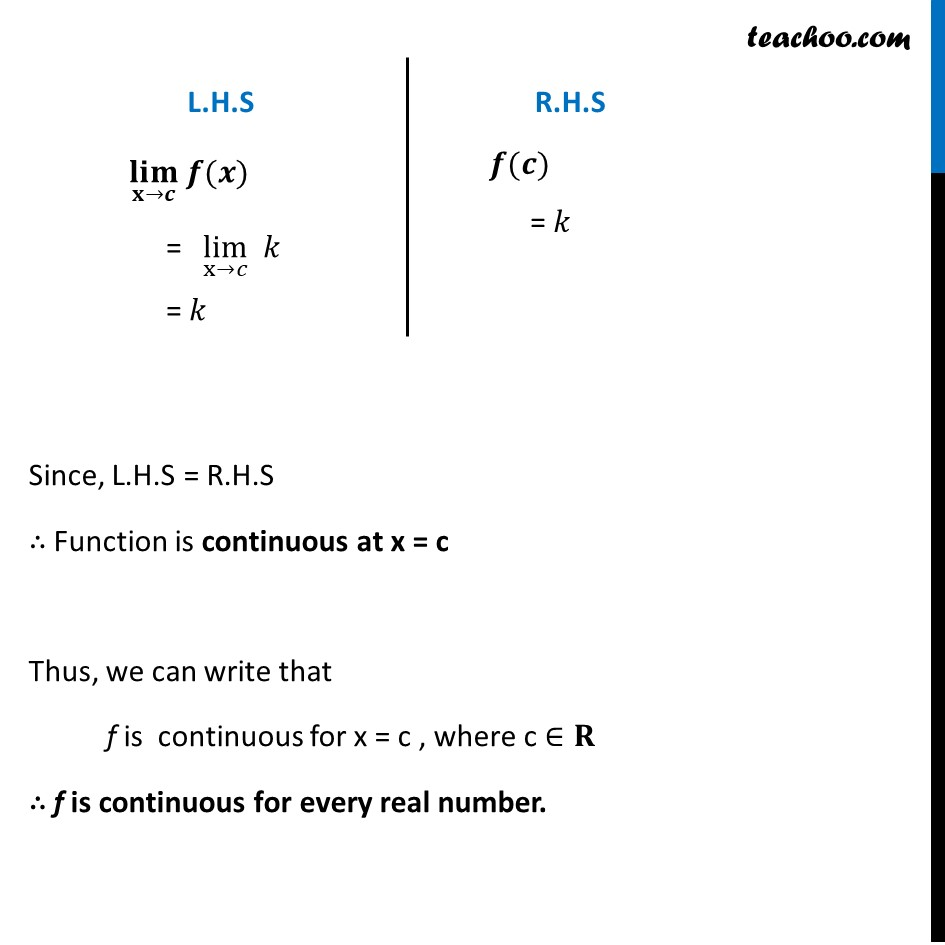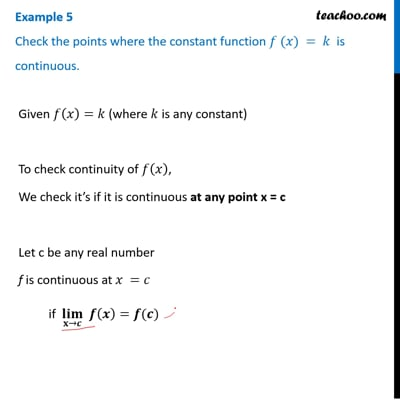Checking continuity at any point

Chapter 5 Class 12 Continuity and Differentiability
Concept wiseThis video is only available for Teachoo black users

Introducing your new favourite teacher - Teachoo Black, at only ₹83 per month

### Transcript

Example 5 Check the points where the constant function 𝑓 (𝑥) = 𝑘 is continuous. Given 𝑓(𝑥)=𝑘 (where 𝑘 is any constant) To check continuity of 𝑓(𝑥), We check it’s if it is continuous at any point x = c Let c be any real number f is continuous at 𝑥 =𝑐 if (𝐥𝐢𝐦)┬(𝐱→𝒄) 𝒇(𝒙)=𝒇(𝒄) (𝐥𝐢𝐦)┬(𝐱→𝒄) 𝒇(𝒙) "= " lim┬(x→𝑐) " " 𝑘 = 𝑘 𝒇(𝒄) = 𝑘 Since, L.H.S = R.H.S ∴ Function is continuous at x = c Thus, we can write that f is continuous for x = c , where c ∈𝐑 ∴ f is continuous for every real number.